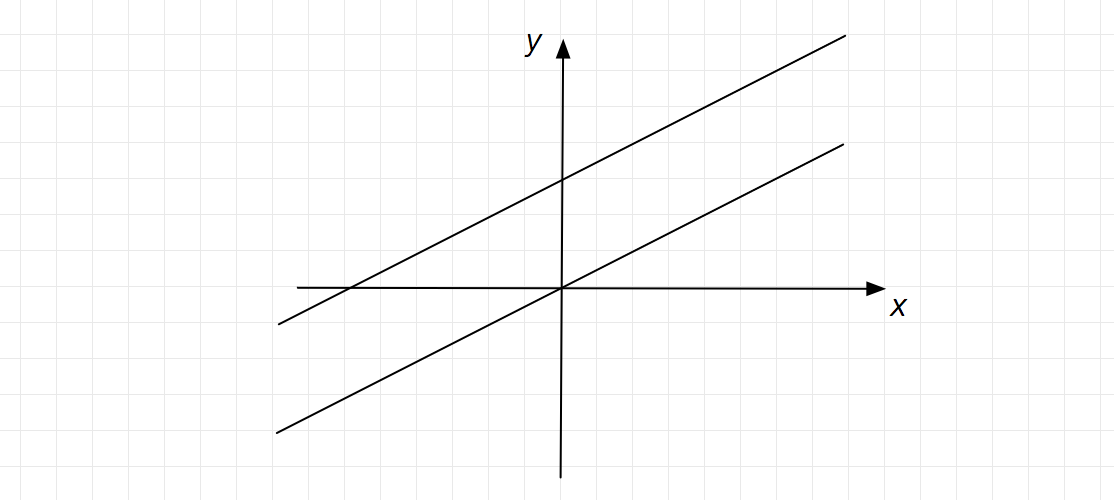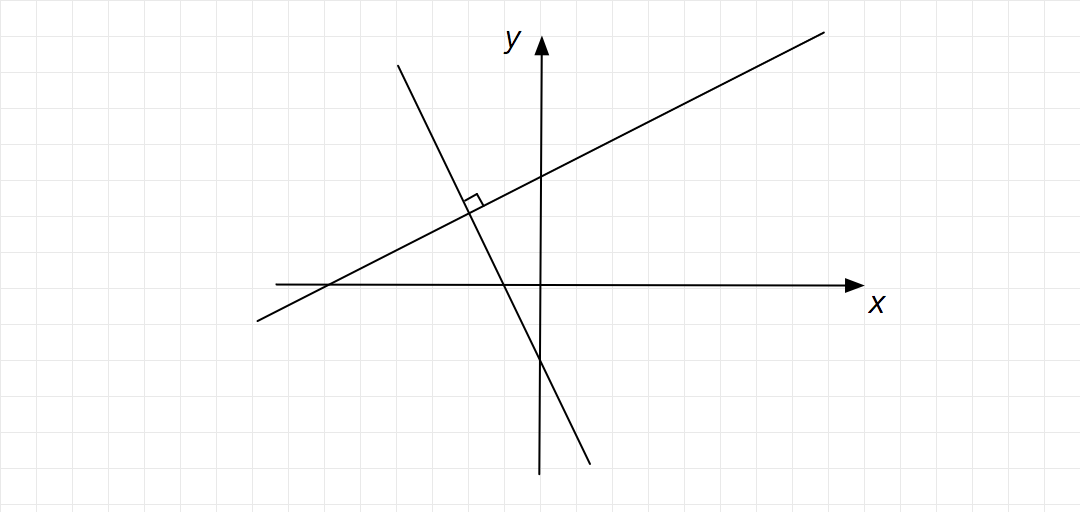Consider the following equations of straight lines:

$\text{Line L1}: 2x – 3y = 5$

$\text{Line L2}: 3x + 2y = 8$

$\text{Line L3}: 4x – 6y = 5$

$\text{Line L4}: 6x – 9y = 6$

Which one among the following is the correct statement?

1. $\text{L1}$ is parallel to $\text{L2}$ and $\text{L1}$ is perpendicular to $\text{L3}$
2. $\text{L2}$ is parallel to $\text{L4}$ and $\text{L2}$ is perpendicular to $\text{L1}$
3. $\text{L3}$ is perpendicular to $\text{L4}$ and $\text{L3}$ is parallel to $\text{L2}$
4. $\text{L4}$ is perpendicular to $\text{L2}$ and $\text{L4}$ is parallel to $\text{L3}$

Given that,

• $2x – 3y = 5 \quad \longrightarrow (1)$
• $3x + 2y = 8 \quad \longrightarrow (2)$
• $4x – 6y = 5 \quad \longrightarrow (3)$
• $6x – 9y = 6 \quad \longrightarrow (4)$

From equation $(1),$

$2x – 3y = 5$

$\Rightarrow -3y = -2x + 5$

$\Rightarrow y = \frac{2}{3}x – \frac{5}{3}$

Slope of the line, ${\color{Blue}{\boxed{m_{1} = \frac{2}{3}}}}$

From equation $(2),$

$3x + 2y = 8$

$\Rightarrow 2y = -3x + 8$

$\Rightarrow y = \frac{-3}{2}x + 4$

Slope of the line, ${\color{Purple}{\boxed{m_{2} = \frac{-3}{2}}}}$

From equation $(3),$

$4x – 6y = 5$

$\Rightarrow -6y = -4x + 5$

$\Rightarrow y = \frac{2}{3}x - \frac{5}{6}$

Slope of the line, ${\color{DarkOrange}{\boxed{m_{3} = \frac{2}{3}}}}$

From equation $(4),$

$6x – 9y = 6$

$\Rightarrow -9y = -6x + 6$

$\Rightarrow y = \frac{2}{3}x – \frac{2}{3}$

Slope of the line, ${\color{Teal}{\boxed{m_{4} = \frac{2}{3}}}}$

We get, ${\color{Red}{\boxed{m_{1} = m_{3} = m_{4} = \frac{2}{3}}}}$

The lines $\text{L1, L3, and L4}$ are parallel to each other.

Now,

• $m_{1} \times m_{2} = \frac{2}{3} \times \frac{-3}{2} = -1$
• $m_{3} \times m_{2} = \frac{2}{3} \times \frac{-3}{2} = -1$
• $m_{4} \times m_{2} = \frac{2}{3} \times \frac{-3}{2} = -1$

The lines $\text{L1, L3, and L4}$ are perpendicular to $\text{L2}.$

${\color{Magenta}{\textbf{Second Method:}}}$

The line $\text{L1}: y = \frac{2}{3}x – \frac{5}{3}$

Slope of the line, ${\color{Blue}{\boxed{m_{1} = \frac{dy}{dx} = \frac{2}{3}}}}$

The line $\text{L2}: y = \frac{-3}{2}x + 4$

Slope of the line, ${\color{Purple}{\boxed{m_{2} = \frac{dy}{dx} = \frac{-3}{2}}}}$

The line $\text{L3}: y = \frac{2}{3}x - \frac{5}{6}$

Slope of the line, ${\color{DarkOrange}{\boxed{m_{3} = \frac{dy}{dx} = \frac{2}{3}}}}$

The line $\text{L4}:y = \frac{2}{3}x – \frac{2}{3}$

Slope of the line, ${\color{Teal}{\boxed{m_{4} = \frac{dy}{dx} = \frac{2}{3}}}}$

Correct Answer $:\text{D}$

${\color{Red}{\textbf{PS:}}}$  The general form of line given by $y = mx + c;$ where $m$ is the slope and $c$ is the intercept on $y$-axis.

${\color{Lime}{\textbf{Parallel lines:}}}$ Parallel lines are lines that do not meet at any point in the $xy$-plane. Another way to characterize parallel lines are distinct lines with the same slope. Suppose we are given two non-vertical lines in slope-intercept form:

\begin{aligned} y &= m_1 x + b_1\\ y &= m_2 x + b_2. \end{aligned}

$\text{The two lines are parallel if} \; {\color{Purple}{\boxed{m_1 = m_2​, \;\text{and}\; b_1 \ne b_2.}}}$${\color{Olive}{\textbf{Perpendicular Lines:}}}$ A pair of lines is perpendicular if the lines meet at $90^\circ$ angle. Given two non-vertical lines in slope-intercept form

\begin{aligned} y &= m_1 x + b_1\\ y &= m_2 x + b_2, \end{aligned}​

The two lines are perpendicular if ${\color{Teal}{\boxed{m_1 = - \frac{1}{m_2} \Rightarrow m_{1} \times m_{2} = -1}}}$​, that is, if the slopes are negative reciprocals of each other.${\color{Magenta}{\textbf{Finding slope using differentiation:}}}$  For a line of the form (or any other form) $y=mx+c,$ we can find its slope by simply taking any two values of $x, {x}_{1}$ and ${x}_{2},$ and their respective $y$ values, ${y}_{1}$​ and ${y}_{2}$​. We find the slope by the formula $\tan \theta =\dfrac {{y}_{1}-{y}_{2}}{{x}_{1}- {x}_{2}}$. In the case of curves our approach is somewhat different. In the above case, we had $\Delta y={y}_{1}-{y}_{2}$​ and $\Delta x={x}_{1}-{x}_{2}$. Now we need to find the slope of tangent to a curve at some point. To do this we again need

$$\tan \theta =\frac {{y}_{1}-{y}_{2}}{{x}_{1}- {x}_{2}},$$

but this time $\Delta y$ and $\Delta x$ tend to zero, which means the interval is very small because it is a tangent at a point.Notice that as the colored pairs of $x_1$​ and $x_2$​ come closer, the tangent shifts to a point on the graph.

When this happens we replace

$$\frac { \Delta y }{ \Delta x } \quad \text{by}\quad \frac { dy }{ dx }$$​

12.0k points